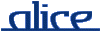alice library manual.The PROBLEM signature

________ Synopsis ____________________________________________________

signature PROBLEM

A PROBLEM is a specification of a constraint problem, including recomputation policy, debug level, and branch and bound optimization. A structure with this signature can be passed as an argument to the MkSearch functor.

Have a look at the examples below.

________ Import ______________________________________________________

import signature PROBLEM from "x-alice:/lib/gecode/search-factory/PROBLEM-sig"

________ Interface ___________________________________________________

signature PROBLEM =
sig
type solution
type space

val root         : space
val readSolution : space -> solution
val copyq        : int -> bool

val bab          : bool
val bound        : space * solution -> unit
val compare      : solution * solution -> bool

end

________ Description _________________________________________________

type solution

The type of concrete solutions. Usually a tuple or a vector of integers.

type space

The type of constraint spaces. Always Space.space.

root

The root space, that is, a fresh constraint space where constraints have been posted. The search algorithm (see SEARCH) will use this space as a starting point.

This function should extract a solution from a solved space (space).

copyq depth

Recomputation policy: all the nodes of the search tree whose depth satisfies the predicate copyq depth = true will be copied and used for recomputation. As an example, a fixed distance recomputation policy can be defined using fun copyq d = d mod fixedDistance = 0, where fixedDistance is a given integer. Note that although this scheme is quite flexible, it is not possible to define real adaptative policies that way.

A debug mask that selects which debug messages are to be printed. See Debug.

bab

Should be set to true for Branch & Bound search. Otherwise (simple search), should be false.

bound (space, solution)

Should bound the given space with the given solution.

Required: This function is called only if Branch & Bound is active (that is, bab = true). Otherwise, you may define it as fun bound _ = assert false.

compare (solution1, solution2)

Should return true if and only if solution2 is better than solution1.

Required: This function is called only if Search.init or Search.betterThan are to be called. It is also used by distributed search with Branch & Bound active. In all other cases, including local search, and local Branch & Bound search, this function is not required and can be defined as fun compare _ = assert false.

________ Examples ____________________________________________________

The first example is the legacy n-queen problem. It uses a fixed distance recomputation policy. It does not use Branch & Bound, thus the corresponding functions are defined as assert false.

import structure Debug     from "x-alice:/lib/gecode/search-factory/Debug"
import structure MkSearch  from "x-alice:/lib/gecode/search-factory/MkSearch"
import structure Space     from "x-alice:/lib/gecode/Space"
import structure FD        from "x-alice:/lib/gecode/FD"
import structure Inspector from "x-alice:/lib/tools/Inspector"

(* The initial root space. *)
val root = Space.new ()

val size = 8

open FD
val cn = FD.BND
val v = rangeVec(root, size, (0, size-1))
val v1 = Vector.tabulate (size, fn i => (i, Vector.sub(v,i)))
val v2 = Vector.tabulate (size, fn i => (~i, Vector.sub(v,i)))

val _ =
(distinctOffset(root, v1, cn);
distinctOffset(root, v2, cn);
distinct(root, v, cn);
branch(root, v, B_SIZE_MIN, B_MIN))

fun toInt space v = FD.Reflect.value (space,v)
fun readSolution space = Vector.map (fn var => FD.Reflect.value (space, var)) v

(* Recomputation Policy : fixed distance *)
val rdist = 3

structure Problem =
struct
type solution = int Vector.t
type space = Space.space

val root = root
fun copyq d = d mod rdist = 0

val bab = false
fun bound _ = assert false
fun compare _ = assert false

end

structure Search = MkSearch Problem

val solutions = Search.getAllSolutions ()

val _ = Inspector.inspect solutions

The second example can be described as follows: given two tuples of n integers, written Ai and Bi, create a new tuple Ci of distinct integers such that for all i, Ci is either Ai or Bi. A score is associated to each solution by computing the sum of the integers of the tuple. Greatest score is best.

import structure Debug     from "x-alice:/lib/gecode/search-factory/Debug"
import structure MkSearch  from "x-alice:/lib/gecode/search-factory/MkSearch"
import structure Space     from "x-alice:/lib/gecode/Space"
import structure FD        from "x-alice:/lib/gecode/FD"
import structure Inspector from "x-alice:/lib/tools/Inspector"

(* The initial root space. *)
val root = Space.new ()

(*** Search problem :
*   Choose one number in each column (numbers1, numbers2)
*   All numbers must be different
*)
val max = 10
val size = 6
val numbers1 = #[2, 1, 2, 5, 1, 6]
val numbers2 = #[1, 3, 4, 3, 6, 7]

infix %
fun a % b = Vector.sub (a,b)

val cn = FD.BND

fun fromInt sp n = FD.intvar (sp,#[(n,n)])
fun toInt   sp v = FD.Reflect.value (sp,v)

val vars  = FD.rangeVec   (root, size, (0, max))
val reif  = FD.boolvarVec (root, size)
val nreif = FD.boolvarVec (root, size) (* means "logical-not of reif" *)
val reif2 = Vector.map FD.boolvar2intvar reif
val sum   = FD.intvar (root, #[(0, size*max)])
val kvars = Vector.tabulate
(size+1, (fn i => if i e+s) 0 sol
val vsum = fromInt space lsum
in
FD.rel (space, sum, FD.GR, vsum)
end

val _ =
(* Propagators. *)
(FD.distinct (root, vars, cn) ;
VectorPair.app
(fn (b1, b2) => FD.nega(root, b1, b2)) (reif, nreif) ;

Vector.appi
(fn (i, var) =>
(FD.Reified.rel
(root, var, FD.EQ, fromInt root (numbers1%i), reif%i) ;
FD.Reified.rel
(root, var, FD.EQ, fromInt root (numbers2%i), nreif%i)))
vars ;

(* Sum *)
FD.linear (root, kvars, FD.EQ, 0, cn) ;

(* Branching policy *)
FD.branch (root, reif2, FD.B_NONE, FD.B_MIN))

(* Recomputation Policy : fixed distance *)
val rdist = 3

structure Problem =
struct
type solution = int Vector.t
type space = Space.space

val root = root
fun copyq d = d mod rdist = 0
val bab = true
val bound = bound

fun compare _ = assert false